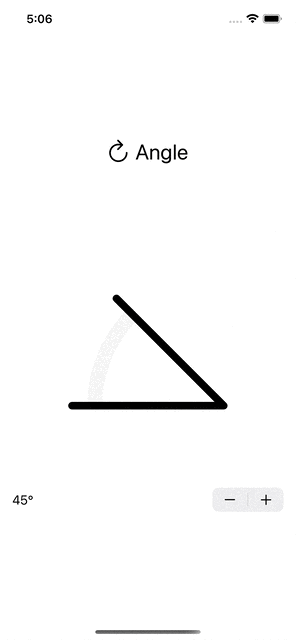# Aim

Last time I showed how I had written a control for inputting an angle. This time I am improving the look of the control as well as adding touch input.# From CGFloat to Angle

But first I want to make an improvement to the code. Now the angle is stored as a `CGFloat` value with convenience functions to convert between radians and degrees. However, this means that it is up to me to remember in which unit the value is at any given moment. Turns out SwiftUI has a struct that solves this: `Angle`. You instantiate it with `Angle.degrees(90)` or `Angle.radians(.pi)` and you can ask for the angle in either unit with `.degrees` and `.radians`.

# Making Angle animatable

Now however, things get a bit weird. Sadly the animation has stopped working. Tapping on the stepper makes the line jump instead of animating. Turns out that while `Angle` conforms to the `Animatable` protocol itself, it doesn’t conform to the `VectorArithmetic` protocol. This is needed for the `Shape` to be animatable. Conforming to `VectorArithmetic` also means conforming to `AdditiveArithmetic`.

Luckily it is simple enough to implement.

``````extension Angle: VectorArithmetic {
public mutating func scale(by rhs: Double) {
}

public var magnitudeSquared: Double {
}
}

public static var zero: Self { .radians(0) }

public static func + (lhs: Self, rhs: Self) -> Self {
}

public static func += (lhs: inout Self, rhs: Self) {
}

public static func - (lhs: Self, rhs: Self) -> Self {
}

public static func -= (lhs: inout Self, rhs: Self) {
}
}
``````

And just by adding those two conformances the input animates again!

# Drawing wedge shape

Next I want draw a wedge shape to fill the space between the two lines. To be able to draw this wedge in a different color I need to create a separate shape. Here having the angle in the `Angle` struct is convenient as that can be used in the call to draw a part of an arc.

``````struct WedgeShape: Shape {
var angle: Angle

var animatableData: Angle {
get { angle }
set { angle = newValue }
}

var insets: UIEdgeInsets = .init(top: 100, left: 100, bottom: 100, right: 100)

func path(in rect: CGRect) -> Path {
let insetRect = rect.inset(by: insets)
assert(insetRect.width == insetRect.height, "Rect must be square")

var path = Path()

let center = CGPoint(x: insetRect.maxX, y: insetRect.maxY)
let radius: CGFloat = insetRect.maxX - insetRect.minX

let startAngle = Angle.degrees(-180)
let endAngle = startAngle + angle

path.closeSubpath()

return path
}
}
``````

Now I have two shapes: `WedgeShape` and `AngleShape` which can each have the desired fill or stroke colors and be stacked on top of each other using a `ZStack`.

Having an input control that doesn’t take touch isn’t much of a control. Next up is adding the ability to use your finger to drag the angle.

A `DragGesture` can facilitate this. When the drag gesture recognizes a change I set the variable `isEditing` to true so that the view can show the user it is being manipulated. And more importantly, the new angle is calculated from the point of rotation to where the user touches the control. Here I am using `atan2` which takes care of the division so you don’t have to worry about dividing by zero.

When the drag ends, the variable `isEditing` should be false again.

`````` @State var isEditing: Bool = false

var dragGesture: some Gesture {
DragGesture()
.onChanged { value in
isEditing = true
let corner = CGPoint(x: 300, y: 300)
let deltaX = corner.x - value.location.x
let deltaY = corner.y - value.location.y
let dragAngle = atan2(deltaY, deltaX)

}
.onEnded { value in
isEditing = false
}
}
``````

Now I am ready to add the gesture to the `AngleView` using the view modifier `.gesture(dragGesture)`.

Now, while this works, it doesn’t feel very responsive. The UI feels laggy. This is because the change in angle is animated, so when changing the angle from the drag gesture animations should be disabled. To achieve this I wrapped the line where the angle is set in a `Transaction` which has animations disabled.

``````var transaction = Transaction(animation: .none)
transaction.disablesAnimations = true

withTransaction(transaction) {
}
``````

# Editing mode

To let the user know the control is being edited I pass the value of the `isEditing` var to the `AngleShape` and draw a circle at the end of the path.

``````if isEditing {
let editCircleRect = CGRect(origin: CGPoint(x: x - 10, y: y - 10), size: CGSize(width: 20, height: 20))